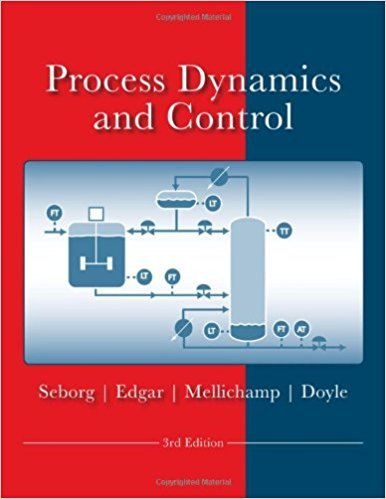×
Get Full Access to Process Dynamics And Control - 3 Edition - Chapter 2 - Problem 2.13
Get Full Access to Process Dynamics And Control - 3 Edition - Chapter 2 - Problem 2.13

×

# The liquid storage tank shown in Fig. E2.13 has two inletISBN: 9780470128671 148

## Solution for problem 2.13 Chapter 2

Process Dynamics and Control | 3rd Edition

• Textbook Solutions
• 2901 Step-by-step solutions solved by professors and subject experts
• Get 24/7 help from StudySoup virtual teaching assistantsProcess Dynamics and Control | 3rd Edition

4 5 1 250 Reviews
18
0
Problem 2.13

The liquid storage tank shown in Fig. E2.13 has two inlet streams with mass flow rates w1 and w2 and an exit stream with flow rate w3. The cylindrical tank is 2.5 m tall and 2 m in diameter. The liquid has a density of 800 kg/m3. Normal operating procedure is to fill the tank until the liquid level reaches a nominal value of 1.75 m using constant flow rates: w1 = 120 kg/min, w2 = 100 kg/min, and w3 = 200 kg/min. At that point, inlet flow rate w1 is adjusted so that the level remains constant. However, on this particular day, corrosion of the tank has opened up a hole in the wall at a height of 1 m, producing a leak whose volumetric flow rate q4 (m3/min) can be approximated by where h is height in meters. (a) If the tank was initially empty, how long did it take for the liquid level to reach the corrosion point? (b) If mass flow rates w1, w2, and w3 are kept constant indefinitely, will the tank eventually overflow? Justify your answer.

Step-by-Step Solution:
Step 1 of 3

Step 2 of 3

Step 3 of 3

##### ISBN: 9780470128671

Unlock Textbook Solution